NEET  >  Speed of a Travelling Wave & Equation of Progressive Wave

# Speed of a Travelling Wave & Equation of Progressive Wave - Notes | Study Physics Class 11 - NEET

 1 Crore+ students have signed up on EduRev. Have you?

Speed of Transverse Motion

On a stretched string v = √(T/m)

where, T = tension in the string and m = mass per unit length of the string.

Speed of transverse wave in a solid v = √(η/ρ)

where, η is modulus of rigidity and ρ is density of solid.

Plane Progressive Simple Harmonic Wave

Equation of a plane progressive simple harmonic wave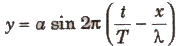or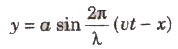where,

• y = displacement,
• a = amplitude of vibration
• λ = wavelength of wave, of particle,
• T = time period of wave,
• x = distance of particle from the origin and
• u = velocity of wave.

Important Relation Related to Equation of Progressive Wave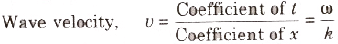where.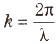ts culled propagation constant of wave motion.
Wavelength,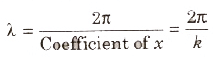Time period.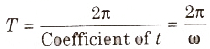Frequency,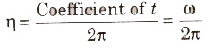Phase of the vibration is the angle of sine m equation, to plan progressive wave. It is denoted by φ.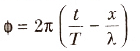Relation between phase difference and path difference and time difference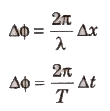The document Speed of a Travelling Wave & Equation of Progressive Wave - Notes | Study Physics Class 11 - NEET is a part of the NEET Course Physics Class 11.
All you need of NEET at this link: NEET

## Physics Class 11

127 videos|464 docs|210 tests
 Use Code STAYHOME200 and get INR 200 additional OFF

## Physics Class 11

127 videos|464 docs|210 tests

### How to Prepare for NEET

Read our guide to prepare for NEET which is created by Toppers & the best Teachers

Track your progress, build streaks, highlight & save important lessons and more!

,

,

,

,

,

,

,

,

,

,

,

,

,

,

,

,

,

,

,

,

,

;